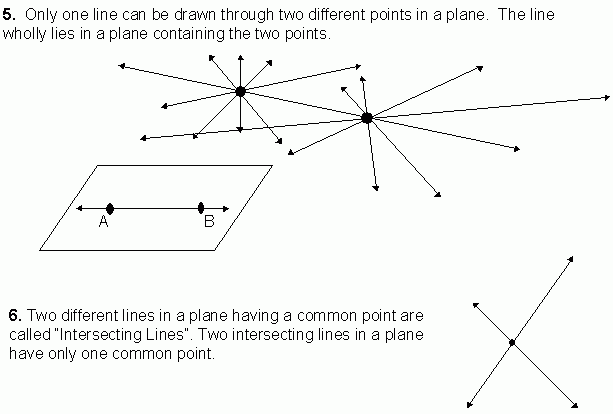Email us to get an instant 20% discount on highly effective K-12 Math & English kwizNET Programs!

#### Online Quiz (WorksheetABCD)

Questions Per Quiz = 2 4 6 8 10

### MEAP Preparation - Grade 7 Mathematics4.29 Basic Geometrical Concepts - Parallel Lines, Collinear Points and PlaneFor videos and additional resources on this topic, please see: MyndBook.com/go.php?to=207_885 Directions: Answer the following questions. Also on a sheet of paper draw a collinear points, non-collinear points and parallel and intersecting lines.
 Q 1: A, B, C and D are four points such that not any three of them are on the same line. Then number of lines that can be drawn through them taking two points at a time is _____.twosixfour Q 2: Number of lines that can be drawn through three non-collinear points, taking two points at a time is _____.twoonethree Q 3: Six points are on a paper such that not any three of them are collinear. Then number of lines that can be drawn joining two points at a time is _____.10515 Q 4: The surface of a box, the cover page of a book etc., are examples of parts of _____.linespointsplanes Question 5: This question is available to subscribers only! Question 6: This question is available to subscribers only!